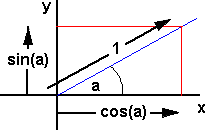# Maths - Rotation Matrices

Rotations can be represented by orthogonal matrices ( there is an equivalence with quaternion multiplication as described here)First rotation about z axis, assume a rotation of 'a' in an anticlockwise direction, this can be represented by a vector in the positive z direction (out of the page).

If we take the point (x=1,y=0) this will rotate to the point (x=cos(a),y=sin(a))

If we take the point (x=0,y=1) this will rotate to the point (x=-sin(a),y=cos(a))

### 3D rotations

For an alterative we to think about using a matrix to represent rotation see basis vectors here.

### Rotation about the z axis

is given by the following matrix:

Rotation about z axis is: Rz =
 cos(a) -sin(a) 0 sin(a) cos(a) 0 0 0 1

For example if we choose an angle of +90 degrees we get

 0 -1 0 1 0 0 0 0 1

the direction of rotation is given by the right hand rulewhere the thumb is in the +z direction (toward the viewer) and the fingers show the positive direction of rotation sowill be rotated to.

The conventions used are explained here and the relationship to other standards here.

Other 90 degrees steps are shown here.

### Similarly with rotation about y axis:

Rotation about y axis is: Ry =
 cos(a) 0 sin(a) 0 1 0 -sin(a) 0 cos(a)

For example if we choose an angle of +90 degrees we get

 0 0 1 0 1 0 -1 0 0

the direction of rotation is given by the right hand rulewhere the thumb is in the +y direction (up) and the fingers show the positive direction of rotation sowill be rotated to.

### And rotation about x axis:

Rotation about x axis is: Rx =
 1 0 0 0 cos(a) -sin(a) 0 sin(a) cos(a)

For example if we choose an angle of +90 degrees we get

 1 0 0 0 0 -1 0 1 0

the direction of rotation is given by the right hand rulewhere the thumb is in the +x direction (right) and the fingers show the positive direction of rotation sowill be rotated to.

### General rotation matrix:

Rotation about a general axis is:
 r00 r01 r02 r10 r11 r12 r20 r21 r22

This general rotation can be represented by a combination of the above rotations about Rz, Ry and Rx. As explained below the order of these rotations is important, there are different conventions for which order is assumed, as explained here.

Rotation matrices are orthogonal as explained here.

## isRotationMatrix

This code checks that the input matrix is a pure rotation matrix and does not contain any scaling factor or reflection for example

```/**
*This checks that the input is a pure rotation matrix 'm'.
* The condition for this is:
* R' * R = I
* and
* det(R) =1
*/
public boolean isRotationMatrix(matrix m) {
double epsilon = 0.01; // margin to allow for rounding errors
if (Math.abs(m*m + m*m + m*m) > epsilon) return false;
if (Math.abs(m*m + m*m + m*m) > epsilon) return false;
if (Math.abs(m*m + m*m + m*m) > epsilon) return false;
if (Math.abs(m*m + m*m + m*m - 1) > epsilon) return false;
if (Math.abs(m*m + m*m + m*m - 1) > epsilon) return false;
if (Math.abs(m*m + m*m + m*m - 1) > epsilon) return false;
return (Math.abs(det(m)-1) < epsilon);
// det is defined here:
// https://www.euclideanspace.com/maths/algebra/matrix/functions/determinant/threeD/
}```

### Successive Rotations

The order of Successive Rotations is significant, for example

1. Rotate 90 degrees about x axis
2. Rotate 90 degrees about y axis
3. Rotate -90 degrees about x axis

This gives 90 degree rotation about Z axis,

whereas

1. Rotate 90 degrees about x axis
2. Rotate -90 degrees about x axis
3. Rotate 90 degrees about y axis

This gives 90 degree rotation about y axis (first 2 lines cancel out).

Successive rotations can be calculated by multiplying together the matrices representing the individual rotations. In the same way that the order of rotations are important, the order of matrix multiplication is important.

So with matrix algebra different rules apply than in the algebra of numbers.

In the first example the 3 rotations would be represented by:

 1 0 0 0 0 -1 0 1 0
×
 0 0 -1 0 1 0 1 0 0
×
 1 0 0 0 0 1 0 -1 0
=
 0 -1 0 1 0 0 0 0 1

in the second case the rotations are represented by:

 1 0 0 0 0 -1 0 1 0
×
 1 0 0 0 0 1 0 -1 0
×
 0 0 -1 0 1 0 1 0 0
=
 0 0 -1 0 1 0 1 0 1

## Axis Angle rotation

Any set of successive rotations can be replaced by a single equivalent rotation:The matrix for this rotation is given by:

[R] =
 1 + (1-cos(angle))*(x*x-1) -z*sin(angle)+(1-cos(angle))*x*y y*sin(angle)+(1-cos(angle))*x*z z*sin(angle)+(1-cos(angle))*x*y 1 + (1-cos(angle))*(y*y-1) -x*sin(angle)+(1-cos(angle))*y*z -y*sin(angle)+(1-cos(angle))*x*z x*sin(angle)+(1-cos(angle))*y*z 1 + (1-cos(angle))*(z*z-1)

where:

• x,y and z represent a unit vector

see axis-angle to matrix conversion

Also see discussion here

## Representing Rotation and Translation

If we want to represent rotation and translation using a single matrix we need to use a 4x4 matrix as explained here.

 metadata block see also: Correspondence about this page Book Shop - Further reading. Where I can, I have put links to Amazon for books that are relevant to the subject, click on the appropriate country flag to get more details of the book or to buy it from them.Mathematics for 3D game Programming - Includes introduction to Vectors, Matrices, Transforms and Trigonometry. (But no euler angles or quaternions). Also includes ray tracing and some linear & rotational physics also collision detection (but not collision response). Specific to this page here: